This mathematics ClipArt gallery offers 100 illustrations of United States coins that add up to values from one cent to one dollar.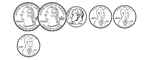### 63 Cents

Groups of change with totals from 1 to 100 cents using the least amount of coins.### 64 Cents

Groups of change with totals from 1 to 100 cents using the least amount of coins.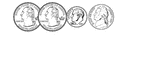### 65 Cents

Groups of change with totals from 1 to 100 cents using the least amount of coins.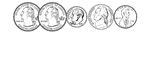### 66 Cents

Groups of change with totals from 1 to 100 cents using the least amount of coins.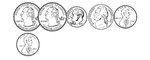### 67 Cents

Groups of change with totals from 1 to 100 cents using the least amount of coins.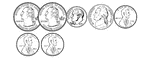### 68 Cents

Groups of change with totals from 1 to 100 cents using the least amount of coins.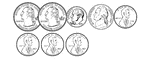### 69 Cents

Groups of change with totals from 1 to 100 cents using the least amount of coins.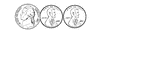### 7 Cents

Groups of change with totals from 1 to 100 cents using the least amount of coins.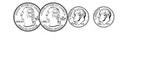### 70 Cents

Groups of change with totals from 1 to 100 cents using the least amount of coins.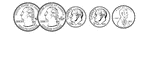### 71 Cents

Groups of change with totals from 1 to 100 cents using the least amount of coins.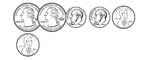### 72 Cents

Groups of change with totals from 1 to 100 cents using the least amount of coins.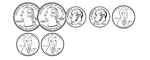### 73 Cents

Groups of change with totals from 1 to 100 cents using the least amount of coins.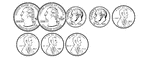### 74 Cents

Groups of change with totals from 1 to 100 cents using the least amount of coins.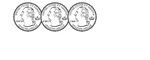### 75 Cents

Groups of change with totals from 1 to 100 cents using the least amount of coins.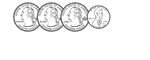### 76 Cents

Groups of change with totals from 1 to 100 cents using the least amount of coins.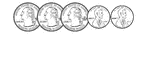### 77 Cents

Groups of change with totals from 1 to 100 cents using the least amount of coins.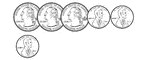### 78 Cents

Groups of change with totals from 1 to 100 cents using the least amount of coins.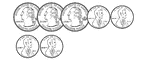### 79 Cents

Groups of change with totals from 1 to 100 cents using the least amount of coins.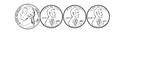### 8 Cents

Groups of change with totals from 1 to 100 cents using the least amount of coins.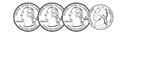### 80 Cents

Groups of change with totals from 1 to 100 cents using the least amount of coins.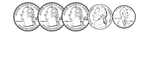### 81 Cents

Groups of change with totals from 1 to 100 cents using the least amount of coins.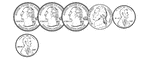### 82 Cents

Groups of change with totals from 1 to 100 cents using the least amount of coins.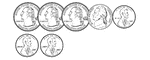### 83 Cents

Groups of change with totals from 1 to 100 cents using the least amount of coins.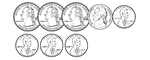### 84 Cents

Groups of change with totals from 1 to 100 cents using the least amount of coins.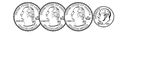### 85 Cents

Groups of change with totals from 1 to 100 cents using the least amount of coins.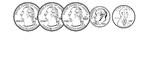### 86 Cents

Groups of change with totals from 1 to 100 cents using the least amount of coins.### 87 Cents

Groups of change with totals from 1 to 100 cents using the least amount of coins.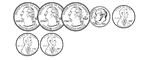### 88 Cents

Groups of change with totals from 1 to 100 cents using the least amount of coins.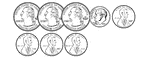### 89 Cents

Groups of change with totals from 1 to 100 cents using the least amount of coins.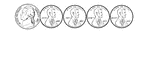### 9 Cents

Groups of change with totals from 1 to 100 cents using the least amount of coins.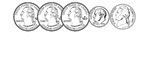### 90 Cents

Groups of change with totals from 1 to 100 cents using the least amount of coins.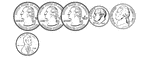### 91 Cents

Groups of change with totals from 1 to 100 cents using the least amount of coins.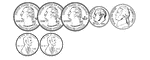### 92 Cents

Groups of change with totals from 1 to 100 cents using the least amount of coins.### 93 Cents

Groups of change with totals from 1 to 100 cents using the least amount of coins.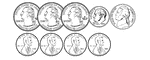### 94 Cents

Groups of change with totals from 1 to 100 cents using the least amount of coins.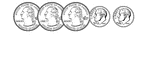### 95 Cents

Groups of change with totals from 1 to 100 cents using the least amount of coins.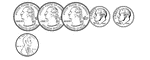### 96 Cents

Groups of change with totals from 1 to 100 cents using the least amount of coins.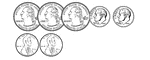### 97 Cents

Groups of change with totals from 1 to 100 cents using the least amount of coins.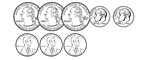### 98 Cents

Groups of change with totals from 1 to 100 cents using the least amount of coins.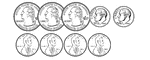### 99 Cents

Groups of change with totals from 1 to 100 cents using the least amount of coins.